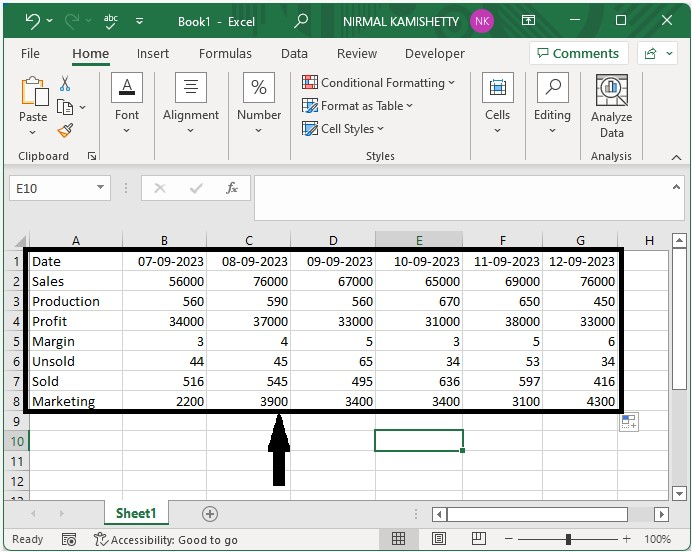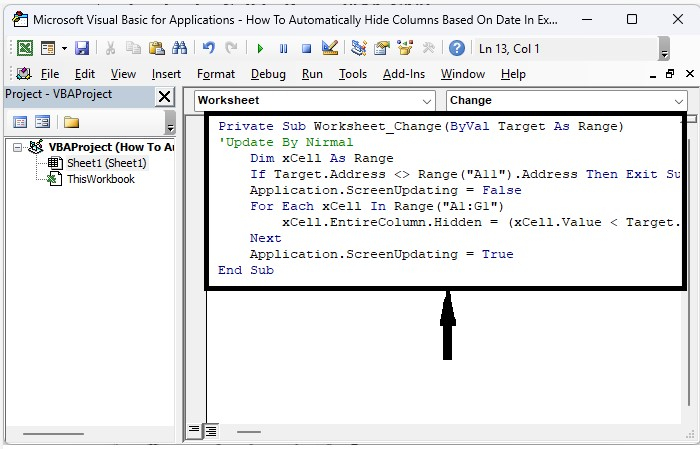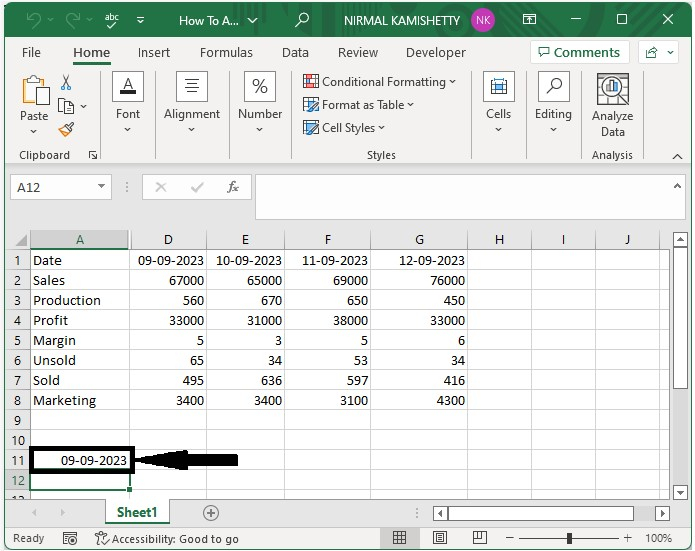# How to Automatically Hide Columns Based on Date in Excel?

Sometimes, when dealing with a list of data, you want to hide a column of data for privacy reasons, but you don't want to delete them because they can be used some other time. In this case, hiding is possible in Excel. We need to use the help of the VBA application, as it cannot be completed directly in Excel. This tutorial will help you understand how we can automatically hide columns based on date in Excel. For example, suppose you want to conceal a day's worth of company records.

## Automatically Hide Columns in Excel Based on Date

Here we will insert VBA code for the sheet to complete the task. Let's take a look at a simple process for automatically hiding columns based on date.

Step 1

Let us consider an Excel sheet where the data is like the data shown in the below workbook.Select the column you want to hide, then right-click on the sheet name and choose View Code to open the VBA application, and then type the programme into the text box as shown in the image below.

### Example

Private Sub Worksheet_Change(ByVal Target As Range)
'Update By Nirmal
Dim xCell As Range
Application.ScreenUpdating = False
For Each xCell In Range("A1:G1")
xCell.EntireColumn.Hidden = (xCell.Value < Target.Value)
Next
Application.ScreenUpdating = True
End Sub


In the code, A11 is the cell we enter the date, and A1:G1 is the range of cells you want to hide.Step 2

Now save the sheet as a macro-enabled template, close the VB application using the command "Alt + Q", close the sheet, and reopen the workbook. Then every time we enter the date in the cell A11, the data belonging to the date less than it will hide automatically, as shown in the below image.## Conclusion

In this tutorial, we used a simple example to demonstrate how you can automatically hide columns based on date in Excel.

Updated on: 10-Jan-2023

1K+ Views